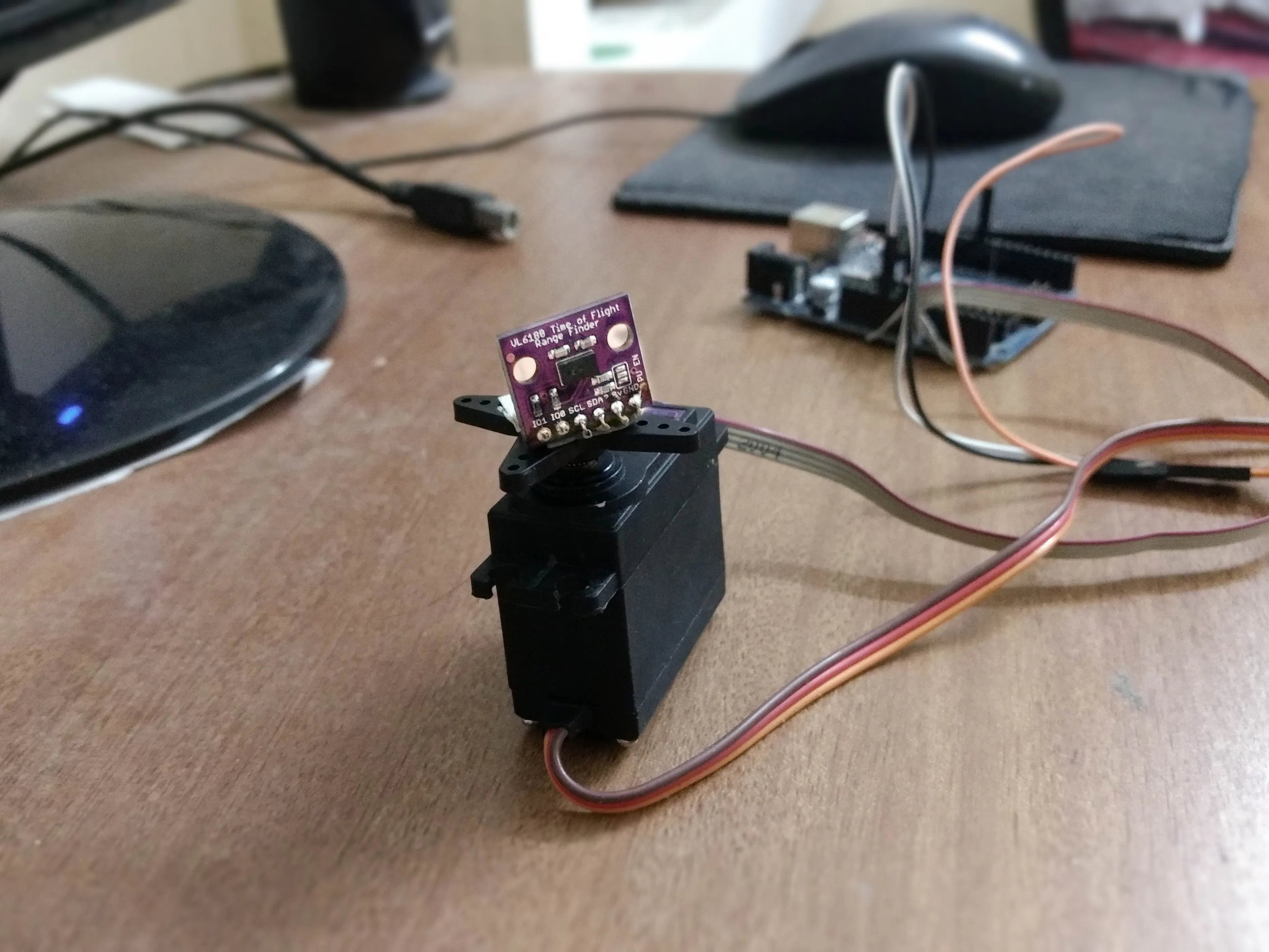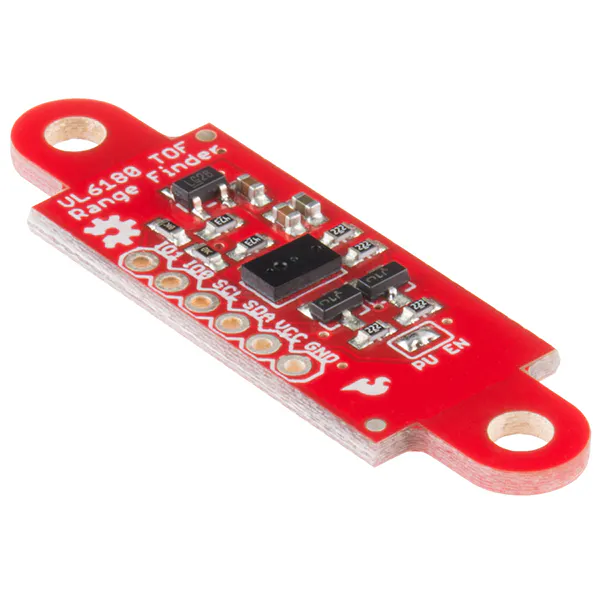# Arduino LIDAR

LIDAR stands for Light Detection and Ranging. LIDAR is used for many applications including 3D scanning, mapping, laser guidance, etc.

IntermediateFull instructions provided1 hour67,103## Things used in this project

### Hardware componentsArduino UNO & Genuino UNO
×1SparkFun ToF Range Finder Sensor - VL6180 VL53L0X for long range and VL6180X for short range. here I used VL6180X
×1
 servo motor
×1

### Software apps and online servicesArduino IDE
 Processing

## Code

### Arduino code

Arduino
```#include <Wire.h>
#include <Servo.h>
Servo myservo;
float pos = 0;
const float Pi = 3.14159;
void setup() {
myservo.attach(9);
Serial.begin(115200);
while (!Serial) {
delay(1);
}

if (! vl.begin()) {
while (1);
}
}

void loop() {

for (pos = 0; pos <= 180; pos += .5) {
myservo.write(pos);
Serial.println(String(range)+"p"+String(pos*Pi/180)+"p"+String(pos));
delay(10);
}
/*for (pos = 180; pos >= 0; pos -= .5) {
myservo.write(pos);
{
Serial.println(String(range)+"p"+String(pos*Pi/180)+"p"+String(pos));
delay(10);
}
}*/
myservo.write(0);
delay(1000);
}
```

### processing code

Processing
```import processing.serial.*;
Serial myPort;
String val;
int range,i=0;float pos;
void setup(){
size(550,500);
frameRate(36);
String portName = "COMx";//x=your arduino port number
myPort = new Serial(this, portName, 115200);
background(255);

}
void draw(){
if ( myPort.available() > 0) {
if(val!=null)
{    String[] nums=split(val,"p");//splitting the recevied data searated by 'p'
if(nums.length==3)
{
range=int(nums); //string to integer conversion
pos=float(nums);
i=int(nums);
if(i==180){
background(255);
}
}
}
}
translate(25,-50);
line(250,500,250-2*(range*cos(pos)),500-2*(range*sin(pos)));
}
```